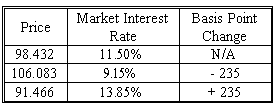### CFA Practice Question

There are 266 practice questions for this study session.

### CFA Practice Question

Shaker Chin has an 11.000%, four year bond that is selling for a price of \$98.432 and is currently yielding 11.50%. The table below shows how the price will vary given a 235 basis point change in market interest rates. Assuming the duration is 3.159 and the convexity is 12.592, what is the total estimated percentage price change based on a 235 BP market rate increase?A. -7.0769%
B. -4.1071%
C. 7.7723%
Explanation: This answer requires candidates to recognize how to handle an interest rate decrease. The duration estimate is a negative adjustment while the positive convexity is a positive adjustment. The detailed solution follows.

Estimated change using duration = Approximate percentage price change = -duration * dy *100, since duration = 3.159 and dy = 0.0235
The estimated change associated with duration = -7.425%

Convexity adjustment = 1/2 * convexity measure * (dy)2 * 100
Since convexity = 12.592, and (dy)2 = (0.0235)2
The adjustment associated with convexity = +0.348%

Total estimated percentage price change = The estimated change associated with duration + estimated change associated with convexity = -7.425% + 0.348% =-7.0769%

Another simple solution is to take prices as given:
(91.466 - 98.432)/98.432 = - 0.070770 = -7.0770%.
However, it remains important to know how to derive the answer using duration and convexity.